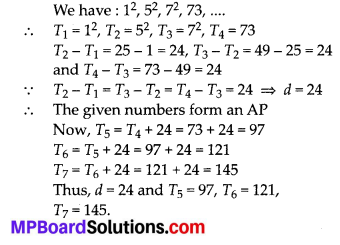In this article, we will share MP Board Class 10th Maths Book Solutions Chapter 5 Arithmetic Progressions Ex 5.1 Pdf, These solutions are solved subject experts from the latest edition books.

## MP Board Class 10th Maths Solutions Chapter 5 Arithmetic Progressions Ex 5.1Question 1.
In which of the following situations, does the list of numbers involved make an arithmetic
progression, and why’
(i) The taxi fare after each km when the fare is ₹ 15 for the first km and 8 for each
(ii) The amount of air present In a cylinder when a vacuum pump removes 1/4 of the
air remaining in the cylinder at a time.
(iii) The cost of digging a well after every metre of digging, when it costs 150 for
the first metre and rises by 50 for each subsequent metre.
(iv) The amount of money in the account every year, when 10000 is deposited at compound interest at 8% per annum.
Solution:
(i) Let us consider, the first term
(T1) Fare for the first 1 km = ₹ 15
Since, the taxi fare beyond the first 1 km is ₹ 8 for each additional km.
∴ Fare for 2 km = ₹ 15 + 1 × ₹ 8
T2 = a + 8 [where a = 15]
Fare for 3 km = ₹ 15 + 2 × ₹ 8
⇒ T3 = a + 16
Fare for 4 km= ₹ 15 + 3 × ₹ 8
⇒ T4 = a + 24
Fare for 5 km = ₹ 15 + 4 × ₹ 8
⇒ T5 = a + 32
Fare for n km = ₹ 15 + (n – 1)8
⇒ Tn = a + (n – 1)8
We see that above terms form an AP with common difference 8.

(ii) Let the amount of air present in the cylinder be x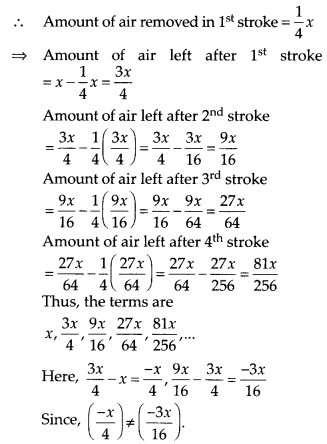The above terms are not in A.P.

(iii) Here, the cost of digging for first 1 metre = ₹ 150
The cost of digging for first 2 metres = ₹ 150 + ₹ 50 = ₹ 200
The cost of digging for first 3 metres = ₹ 150 + (₹ 50) × 2 = ₹ 250
The cost of digging for first 4 metres = ₹ 150 + (₹ 50) × 3 = ₹ 300
∴ The terms are: 150, 200, 250, 300,…..
Since, 200 – 150 = 50 and 250 – 200 = 50
(200 – 150) (250 – 200) = 50
∴ The above terms form an AP with common difference 50.

(iv)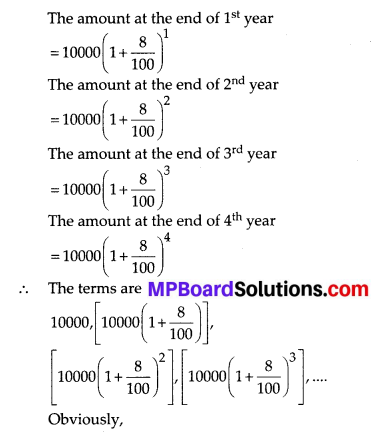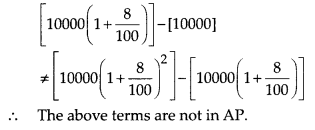Question 2.
Write first four terms of the AP, when the first term a and the common difference d are given
as follows:
(i) a = 10, d = 10
(ii) a = -2, d = 0
(iii) a = 4, d = -3
(iv) a = -1, d = 1/2
(y) a = -1.25, d = -0.25
Solution:
(i) ∵ Tn = a + (n – 1)d
∴ For a = 10 and d = 10, we have:
T1 =10 + (1 – 1) × 10 = 10 + 0 = 10
T2 = 10 + (2 – 1) × 10 = 10 + 10 – 20
T3 = 10 + ( 3 – 1) × 10 = 10 + 20 = 30
T4 = 10 + (4 – 1) × 10 = 10 + 30 = 40
Thus, the first four terms are:
10, 20, 30, 40

(ii) ∵ Tn = a + (n – 1)d
∴ For a = -2 and d = 0,we have:
T1 = -2 + (1 – 1) × 0 = -2 + 0 = -2
T2 = -2 + (2 – 1) × 0 = -2 + 0 = -2
T3 = -2 + (3 – 1) × 0= -2 + 0 = -2
T4 = -2 + (4 – 1) × 0 = -2 + 0 = -2
∴ Thus, the first four terms are: -2, -2, -2, -2

(iii) ∵ Tn = a + (n – 1)d
For a = 4 and d = -3, we have:
T1 = 4 + (1 – 1) × (-3) = 4 + 0 = 4
T2 = 4 + (2 – 1) × (-3) = 4 + (-3) = 1
T3 = 4 + (3 – 1) × (-3) = 4 + (-6) = -2
T4 = 4 + (4 – 1) × (-3) = 4 + (-9) = -5
Thus, the first four terms are:
4, 1, -2, -5

(iv)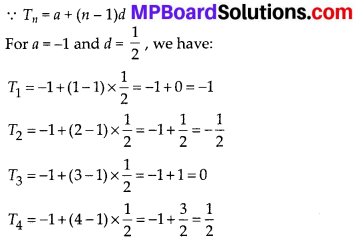(v) ∵ Tn = a + (n – 1)d
∴ For a = -1.25 and d = -0.25, we have
T1 = -1.25 + (1 – 1) × (-0.25) = -1.25 + 0
= -1.25
T2 = -1.25 + (2 – 1) × (-0.25) = -1.25 + (-0.25) = -1.50
T3 = -1.25 + (3 – 1) × (-0.25) = -1.25 + (-0.50) = -1.75
T4 = -1.25 + (4 – 1) × (-0.25) = -1.25 + (-0.75) = -2.0Question 3.
For the following APs, write the first term and the common difference:
(i) 3, 1, -1, -3,…..
(ii) -5, -1, 3, 7,….
(iii) $$\frac{1}{3}, \frac{5}{3}, \frac{9}{3}, \frac{13}{3}, \ldots .$$
(iv) 0.6, 1.7, 2.8, 3.9, …..
Solution:
(i) We have : 3, 1, -1, -3, …
⇒ T1 ⇒ 3 = a = 3
T2 = 1, T3= -1, T4= -3
∴ T2 – T1 = 1 – 3 = -2
T4 = T3 = -3 – (-1) = -3 + 1 = -2 ⇒ d = -2

(ii) We have : -5, -1, 3, 7,….
⇒ T1 = -5 ⇒ a = -5, T2 = -1, T3 = 3, T4 = 7
∴ T2 – T1 = -1 – (-5) = -1 + 5 = 4
and T4 – T3 = 7 – 3 = 4 ⇒ d = 4
Thus a = -5, d = 4

(iii)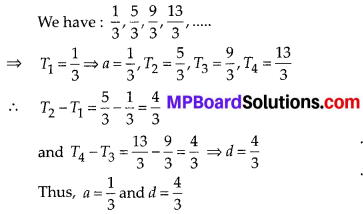Question 4.
Which of the following are APs? If they form an AP, find the common difference d and write three more terms.
(i) 2, 4, 8, 16,
(ii) 2, $$\frac{5}{2}$$, 3, $$\frac{7}{2}$$, ….
(iii) -1.2, -3.2, -5.2, -7.2, ……
(iv) -10, -6, -2, 2, ….
(v) $$3,3+\sqrt{2}, 3+2 \sqrt{2}, 3+3 \sqrt{2}, \ldots$$
(vi) 0.2, 0.22, 0.222, 0.2222, ….
(vii) 0, -4, -8, -12,
(ix) 1, 3, 9, 27, …..
(x) o, 2a, 3a, 4a,…
(xi) a, a2, a3, a4,….
(xii) $$\sqrt{2}, \sqrt{8}, \sqrt{18}, \sqrt{32}, \dots$$
(xiii) $$\sqrt{3}, \sqrt{6}, \sqrt{9}, \sqrt{12}, \dots .$$
(xiv) 12, 32, 52, 72, ….
(xv) 12, 52, 72, 73, …..
Solution:
(i)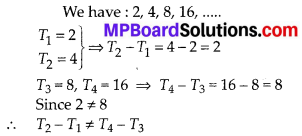∴ The given numbers do not form an AP

(ii)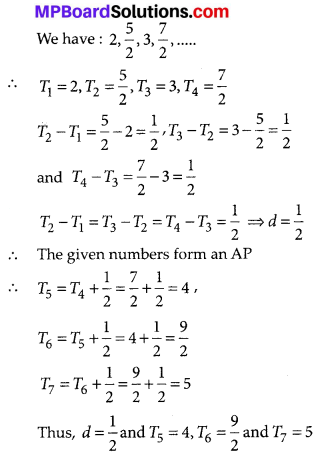(iii) We have : -1.2, -3.2, -5.2, -7.2,
∴ T1 = -1.2, T2 = -3.2, T3 = -5.2, T4 = -7.2
T2 – T1 = -3.2 + 1.2 = -2
T3 – T2 = -5.2 + 3.2 = -2
T4 – T3 = -7.2 + 5.2 = -2
∵ T2 – T1 = T3 – T2 = T4 – T3 = -2 d = -2
∴ The given numbers form an AP such that d = -2.
Now, T5 = T4 + (-2) = -7.2 + (-2) = -9.2,
T6 = T5 + (-2) = -9.2 + (-2) = -11.2 and
T7 = T6 + (-2) = -11.2 + (-2) = -13.2
Thus, d = -2 and T5 = -9.2, T5 = -11.2 and T6 = -13.2

(iv) We have : -10, -6, -2, 2,
T1 = -10, T2 = -6, T3 = -2, T4 = 2
T2 – T1 = -6 + 10 = 4
T3 – T2 = -2 + 6 = 4
T4 – T3 = 2 + 2 = 4
∵ T2 – T1 = T3 – T2 = T4 – T3 = 4 ⇒ d = 4
∴ The given numbers form an AP Now, T5 = T4 + 4 = 2 + 4 = 6,
T6 = T5 + 4 = 6 + 4 = 10,
T7 = T6 + 4 = 10 + 4 = 14
Thus, d = 4 and T5 = 6, T6 = 10, T7 = 14

(v) We have :
3, 3 + $$\sqrt{2}$$, 3 + 2$$\sqrt{2}$$, 3 + 3$$\sqrt{2}$$,….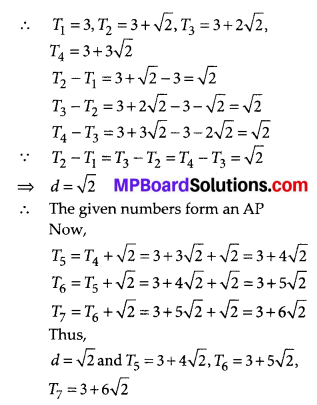(vi) We have : 0.2, 0.22, 0.222, 0.2222,….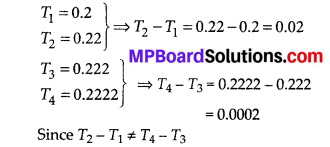∴ The given numbers do not form an AP

(vii) We have : 0, – 4, – 8, – 12,
∴ T1 = 0, T2 = -4, T3 = -8, T4 = -12 T2 – T1 = -4 – 0 = -4
T3 – T2 = – 8 + 4 = – 4
T4 – T3 = -12 + 8 = -4
∴ T2 – T1 = T3 – T2 = T4 – T3 = -4 ⇒ d = -4
∴ The given numbers form an AP
Now, T5 = T4 + (- 4) = -12 + (- 4) = -16
T6 = T5 + (-4) = -16 + (-4)= -20
T7 = T6 + (- 4) = -20 + (- 4) = -24
Thus, d = – 4 and T5 = -16, T6 = -20,
T7 = -24.

(viii)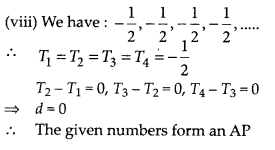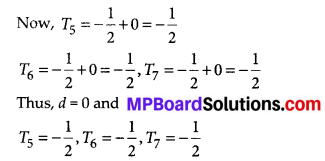(ix) We have, 1, 3, 9, 27,….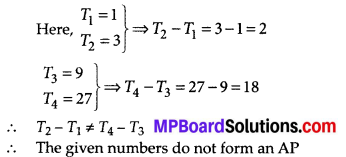(x) We have : a, 2a, 3a, 4a,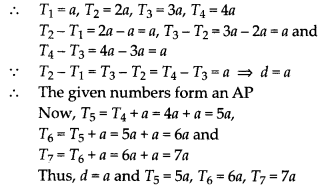(xi)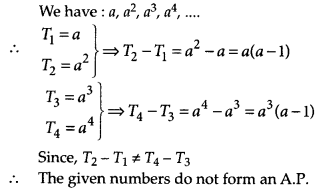(xii)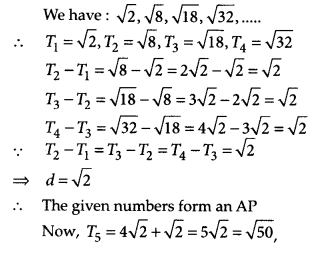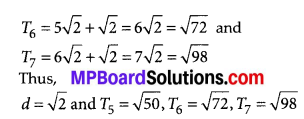(xiii)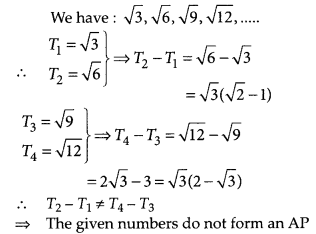(xiv)
We have : 12, 32, 52, 72,….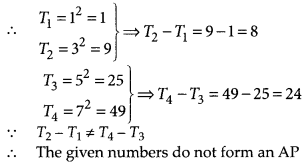(xv) We have : 12 , 52, 72, 72,….